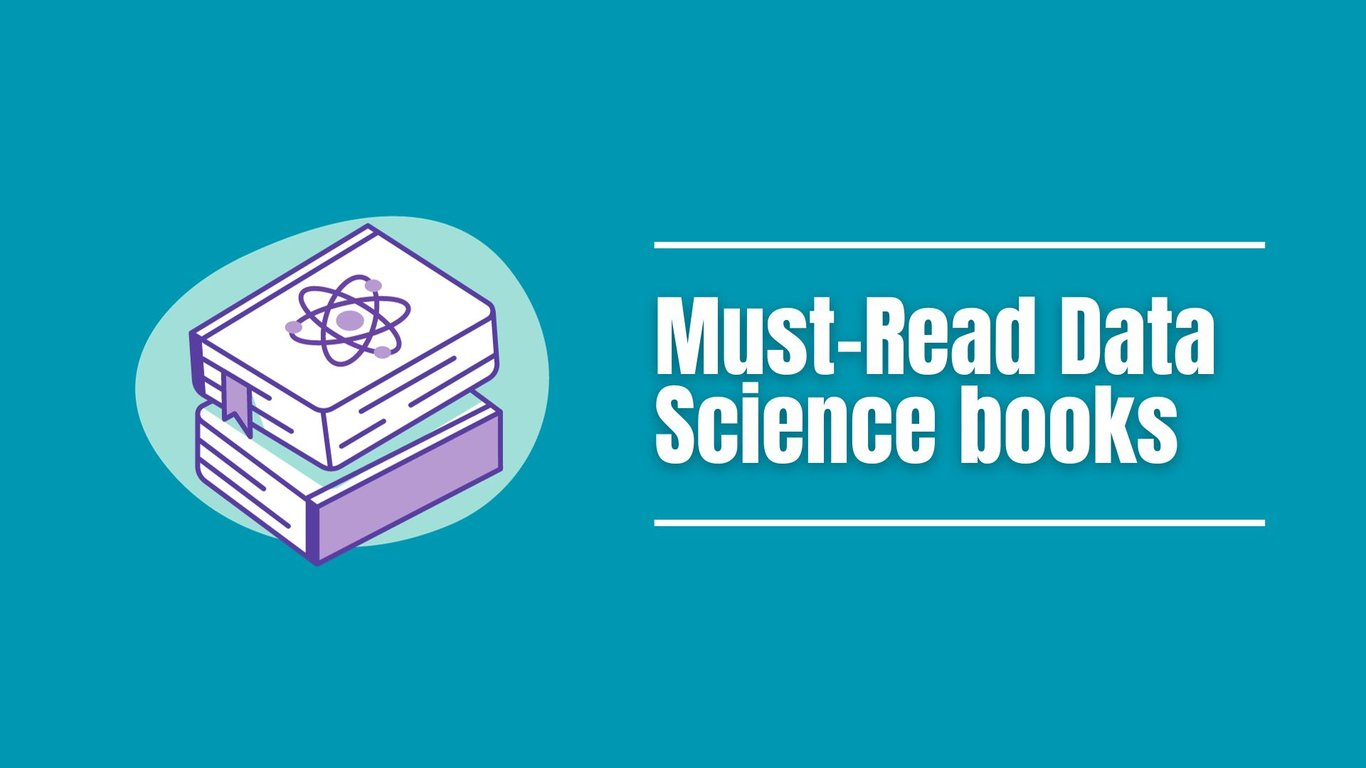# 7 Best Data Science Books For Beginners in 2023Embarking on a journey into the world of data science? Whether you're intrigued by the idea of extracting insights from data or eager to dive into the realm of predictive algorithms, having the right resources at your disposal can make a significant difference. We've compiled a list of seven top-notch data science books for beginners in 2023. These data science books cover a range of topics, from programming and statistics to practical applications of data science techniques.

## 1. Data Science from Scratch: Principles and Python

New to data science? "Data Science from Scratch" is the perfect starting point. This data science book introduces you to the fundamental concepts of data analysis, data visualization, and machine learning using Python. With hands-on examples and clear explanations, you'll gain a solid foundation in data science principles.

## 2. Becoming a Data Head: Understand Statistics

Statistics forms the backbone of data science. "Becoming a Data Head" provides a beginner-friendly introduction to statistical concepts and their applications in the data science field. This data science book demystifies statistical terms and guides you through practical scenarios where statistics play a crucial role.

## 3. Introduction to Data Science and Prediction Algorithms

Curious about prediction algorithms and their real-world applications? "Introduction to Data Science and Prediction Algorithms" offers a comprehensive overview of data analysis, prediction techniques, and their implementation. This data science book helps you understand the underlying principles of making predictions from data.

## 4. Practical Data Science with Python: Hands-On Techniques for Beginners

"Practical Data Science with Python" is a hands-on guide that takes you through the entire data science workflow, from data cleaning and exploration to modeling and visualization. With practical examples and code snippets, this data science book equips you with the skills needed to tackle real-world data science challenges.

## 5. Essential Math for Data Science: Fundamental Theories and Applications

Mathematics is the language of data science. "Essential Math for Data Science" focuses on the mathematical concepts that underlie various data science techniques. Through clear explanations and illustrative examples, this data science book helps you grasp the mathematical foundations of data science.

## 6. Data Science For Dummies

Looking for a comprehensive yet beginner-friendly guide? "Data Science For Dummies" covers a wide range of data science topics, from data exploration and visualization to machine learning and predictive modeling. This data science book breaks down complex concepts into easily digestible explanations.

## 7. Hands-On Introduction to Data Science

"Hands-On Introduction to Data Science" is a practical guide introducing data science concepts through interactive exercises and real-world examples. This data science book helps you build a strong foundation in data analysis, data visualization, and machine learning through active engagement.

Whether you're a complete novice or have a basic understanding of data science, these seven books will provide you with the knowledge and skills needed to kickstart your journey in this exciting field. Remember, the key to mastering data science is continuous learning and hands-on experience. Happy reading and exploring the world of data science!

# Join AI & Bots mailing list

We won't spam. Pinky promise